2nd Grade Word Problems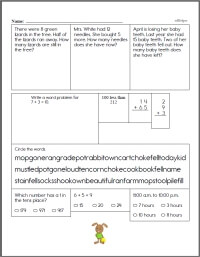Second Grade Word Problems

 3rd Grade Word Problems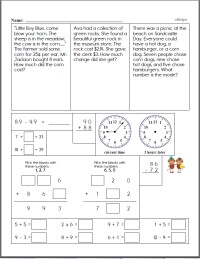Third Grade Word Problems

 4th Grade Word Problems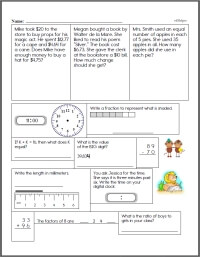Fourth Grade Word Problems

 5th Grade Word Problems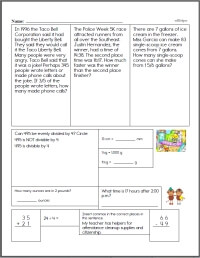Fifth Grade Word Problems

 6th Grade Word Problems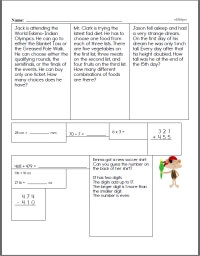Sixth Grade Word Problems

Algebra Word Problems
Algebra Word Problems

Weekly Math Word Problems
This week's first grade math word problems
This week's second grade math word problems
This week's third grade math word problems
This week's fourth grade math word problems
This week's fifth grade math word problems
This week's sixth grade math word problems
This week's middle school math course 1 math word problems
This week's middle school math course 2-3 math word problems

Daily basic skills practice printables: Math, Language Arts, Science, and Math Word Problems
Grade 1 Daily Skills (no science)